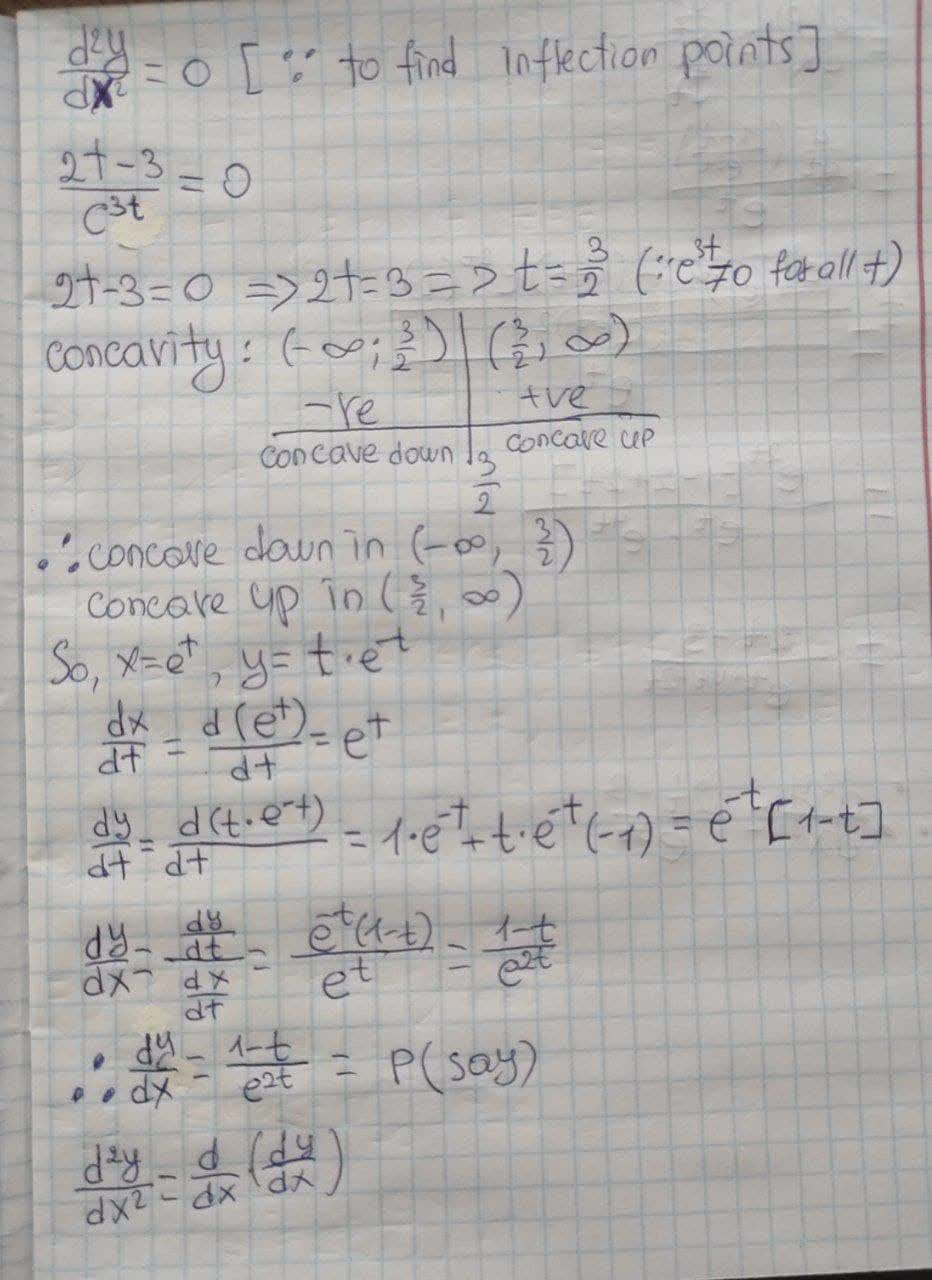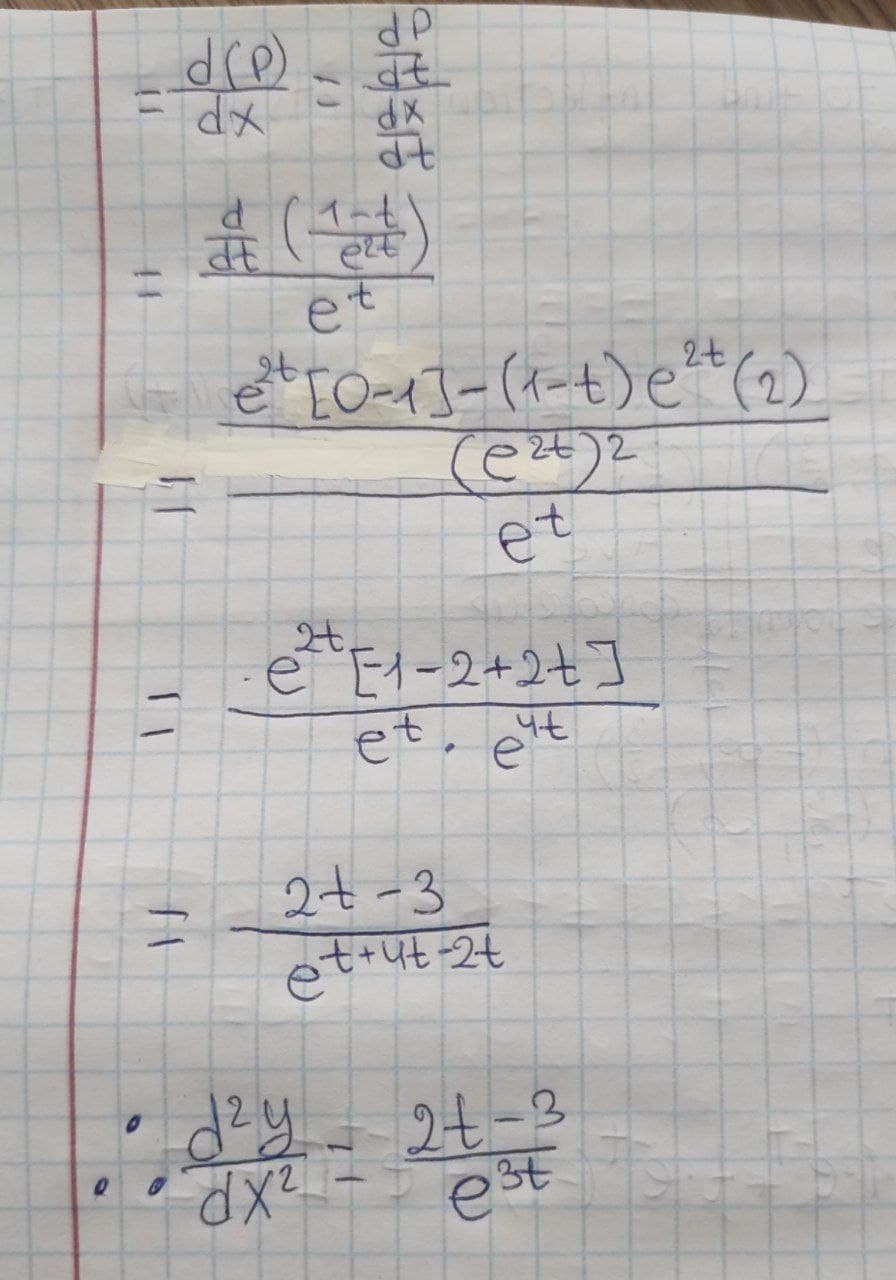# Find dy/dx and d^{2}y/dx^{2}. x = e^{t}, y = te^{-t}. For which values of t is the curve concave upward?chillywilly12a 2021-09-09 Answered

Find $$\displaystyle\frac{{\left.{d}{y}\right.}}{{\left.{d}{x}\right.}}$$ and $$\displaystyle\frac{{{d}^{{{2}}}y}}{{\left.{d}{x}\right.}^{{{2}}}}$$.
$$\displaystyle{x}={e}^{{{t}}},{y}={t}{e}^{{−{t}}}$$
For which values of t is the curve concave upward?

• Questions are typically answered in as fast as 30 minutes

### Plainmath recommends

• Get a detailed answer even on the hardest topics.
• Ask an expert for a step-by-step guidance to learn to do it yourself.Lacey-May Snyder

How to find: